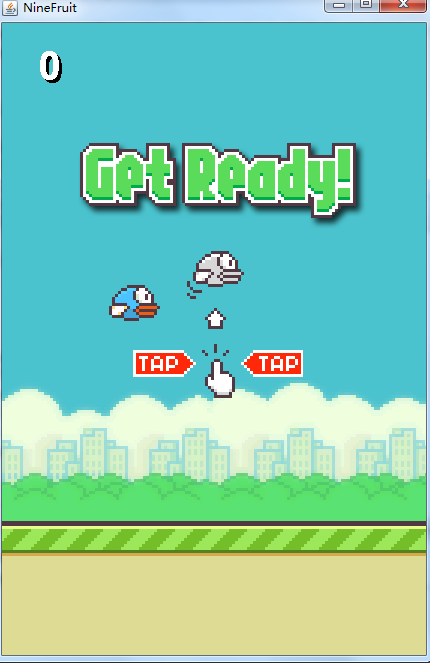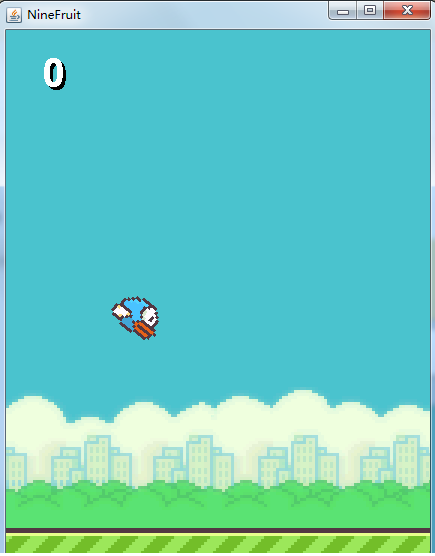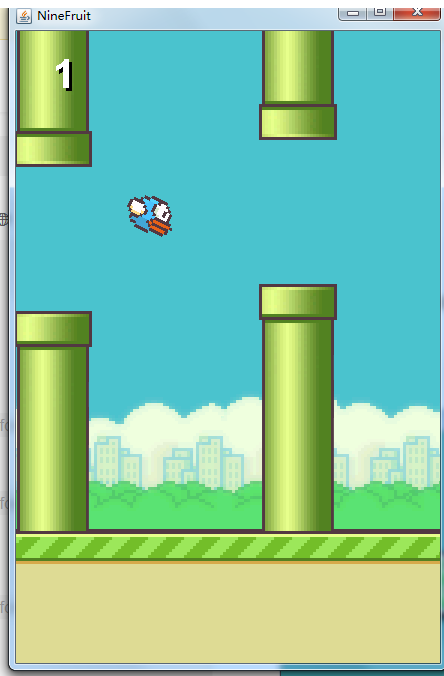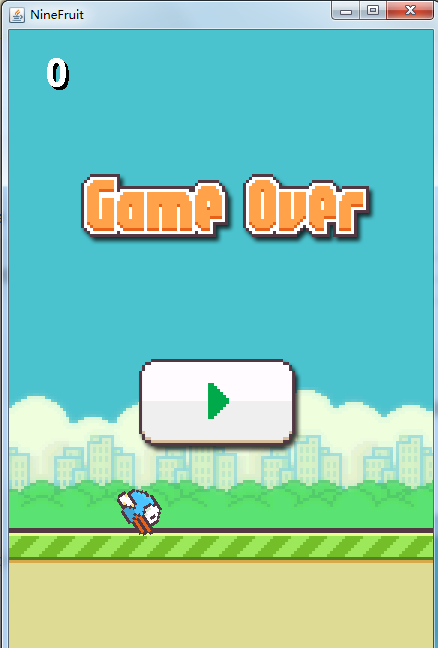# 仿写：《飞翔的小鸟》游戏代码部分

## 代码部分

### 主页类

package cn.ayxy;

import java.awt.Color;
import java.awt.Font;
import java.awt.Frame;
import java.awt.Graphics;
import java.awt.Graphics2D;
import java.awt.Image;
import java.awt.event.MouseEvent;
import java.awt.event.MouseListener;
import java.awt.image.BufferedImage;
import java.io.IOException;
import java.util.concurrent.ForkJoinPool.ManagedBlocker;
import javax.imageio.ImageIO;
import javax.swing.JFrame;
import javax.swing.JPanel;
public class BirdGame extends JPanel{
//启动飞翔的小鸟游戏的方法
/*声明变量*/
Bird bird;//鸟类
Column column1;//柱子类1 前一根
Column column2;//柱子类2 后一根
Ground groud;//地面类
BufferedImage background;//创建BufferedImage对象
int score;//分数
boolean gameOver;//判断是否结束
BufferedImage gameOverImage;//背景图片
//游戏结束属性
int state;//游戏的状态
//公开的权限 （访问权限）  静态不可改变的  常量变量名 一定要大写
public static final int START = 0;//游戏初始状态
public static final int RUNNING = 1;//
public static final int GAME_OVER = 2;//游戏结束状态
//游戏属性图片
BufferedImage stateImage;

public static void main(String[] args) throws Exception {   //有个抛出异常Exception
/*创建一个窗体*/
JFrame frames = new JFrame("NineFruit");//加入字符串 给窗口一个名字
BirdGame game = new BirdGame();//
//设置窗体大小
frames.setSize(440,670);
//设置窗口显示中间
frames.setLocationRelativeTo(null);
//用户关闭窗口时，做的操作
frames.setDefaultCloseOperation(JFrame.EXIT_ON_CLOSE);
/*setDefaultCloseOperation()；是用来设定窗口被关闭时（比如点击了右上角的”x”）的行为。
EXIT_ON_CLOSE：结束窗口所在的应用程序。在窗口被关闭的时候会退出JVM。*/
//设置窗口显示
frames.setVisible(true);
//地面动起来
game.action();
}
//地面移动
public void action()throws Exception{//移动方法 地面
//写一个监听事件
MouseListener l = new MouseAdapter() {
//鼠标按下的方法  void表示没有返回值
@Override
public void mousePressed(MouseEvent e) {
// 获得点击
//bird.flappy();//调用鸟类的飞翔
//抛出异常 抛给 Java编译器 自己处理
try {
switch (state) {
case GAME_OVER://结束状态
//二次赋值  容器
column1 = new Column(1);
column2 = new Column(2);
bird = new Bird();
score = 0;//分数
state = START;
break;
case START://启动状态
//二次赋值  容器
state = RUNNING;
break;
case RUNNING://运行的状态
bird.flappy();//运行调动飞翔方法
break;
}

} catch (Exception e2) {
// TODO: handle exception
e2.printStackTrace();
}
}
};
//将 l 挂接到面板上
while(true){
//                  //什么时候可以飞
//                  if (!gameOver) {//当为false 可以飞  或  gameOver == false
//                      //游戏没有结束的时候飞
//                      groud.step();//重新绘画
//                      column1.step();//移动第一个柱子
//                      column2.step();//移动第二个柱子
//                      bird.step();//鸟移动
//                      bird.fly();//鸟挥动翅膀
//                  }
//                  //判断是否碰撞
//                  if (bird.hlt(groud) || bird.hlt(column1)|| bird.hlt(column2)) {//碰到地面  或者碰到 第一个柱子 和第二个柱子
//                      gameOver = true;//如果碰撞 就真的gameover
//                  }
//
//                  //计分逻辑
//                  if (bird.x == column1.x || bird.x ==  column2.x ) {
//                      score++;//每次分数加
//                  }
//
switch (state) {
case START://启动
groud.step();
bird.fly();//小鸟飞翔状态
break;
case RUNNING://启动
groud.step();
column1.step();
column2.step();
bird.fly();//鸟飞翔动
bird.step();
//积分
if (bird.x == column1.x || bird.x == column2.x ) {
score++;
}
//碰撞
if (bird.hlt(column1) || bird.hlt(column2) || bird.hlt(groud)) {
//碰撞 第一个柱子 或者 第二个柱子 或者 地面
state = GAME_OVER;//游戏状态为结束
}
}
repaint();
//就是1 秒 看到 多少 这是每秒30
}
}
//重写paint 方法  制作一个画笔
public void paint(Graphics g){//这个Graphics 是画笔参数
//绘制背景
g.drawImage(background, 0, 0, null);//最后一个参数  相当于一个检查者  查看背景图片是否改变
/*
* g.drawImage(img, x, y, width, height, observer)
*
*   第一个参数  是背景
*   第二个参数  是横坐标
*   第三个参数  是纵坐标
*   第四个参数  是检查者
*/
//绘制柱子
/*拿第一根柱子的X坐标 减去 第一根柱子的宽2分之1
*拿第一根柱子的Y坐标 减去 第一根柱子的高2分之1
*/
g.drawImage(column1.image, column1.x - column1.width / 2,column1.y - column1.height / 2,null);
g.drawImage(column2.image, column2.x - column2.width / 2, column2.y - column2.height / 2, null);
//绘制地面
g.drawImage(groud.image, groud.x, groud.y, null);
//绘制小鸟
//改一下画笔 API方法 旋转绘图坐标
Graphics2D g2 = (Graphics2D)g;//把之前的画笔强制转换成这个新的  ## g的值不变
//开始反转
//把鸟的X  Y坐标翻转  这个度  有一个向上，向下的动作（变过以后，再变回来）
g2.rotate(-bird.alp, bird.x, bird.y);//负角度 小鸟的头变

//X坐标 减去 宽度的二分子一  *根柱子的坐标一样
g.drawImage(bird.image, bird.x - bird.width / 2, bird.y - bird.height / 2, null);
//再变回来
g2.rotate(bird.alp, bird.x, bird.y);//小鸟的头在变过来
//  里面的三个参数   字体 样式  大小
Font f = new Font(Font.SANS_SERIF, Font.BOLD, 40);//属性 不带括号  方法 带括号
g.setFont(f);
//绘制分数
g.drawString(""+score, 40, 60);//分数转换字符串 X Y 显示位置  做为背景色
g.setColor(Color.WHITE);//字体的颜色为白色
g.drawString(""+score, 40-3, 60-3);
//游戏结束的画面
switch (state) {
case GAME_OVER:
g.drawImage(gameOverImage, 0, 0, null);
break;
case START:
g.drawImage(stateImage, 0, 0, null);
break;
}
}
//添加构造方法，或者构造器，或者构造函数。
/*
* 第一次赋值 叫初始化
* 第二次赋值 是赋值
* 自动调用
* 类是类型 不真实存在 需要对象
*/
public BirdGame() throws Exception{// 抛出异常Exception最大异常
/*
* 这里有一个错误1
* column1 = new Column(1);//这里传参数1
* column2 = new Column(2);//这里传参数2
* 修改 是在 柱子类 里面
*/
/*初始化*/
state = START;//游戏初始状态

bird = new Bird();
groud = new Ground();
column1 = new Column(1);//这里传参数1
column2 = new Column(2);//这里传参数2
//这里是把图片加载进来 添加的是背景图片"图片的名字"
gameOver = false;
}
}


### 小鸟类

package cn.ayxy;

import java.awt.image.BufferedImage;
import java.io.IOException;
import javax.imageio.ImageIO;
import javax.swing.JPanel;

/*  这个是小鸟类
*
* X Y这个是鸟类的中心点坐标
*/
public class Bird extends JPanel{
BufferedImage image;//图片
int x,y,width,height;//横，纵，宽，高
int size;//表示小鸟的大小，用于检测碰撞的使用，表示正方形的边长
//继续增加属性 用于计算鸟的位置
double g;//重力加速度
double t;//两次位置时间的间隔
double v0;//初始上抛速度
double s;//时间t以后的位移
double alp;//弧度，鸟的倾角
double speed;//当前的上抛速度
//定义一个数组 是鸟的动画帧
BufferedImage[] images;
int index;//这个变量是数组元素的下标 从0开始

//构造器
public Bird() throws Exception{//抛出异常 Exception最大异常
width = image.getWidth();
height = image.getHeight();
size = 40;//小鸟的大小
x = 132;//小鸟在屏幕的距离
y = 280;
//初始化 定义的
g = 4;//真实的不是这 是9.4
v0 = 20;//初速度
t = 0.25;//两次位置的时间隔
speed = v0;//当前的上抛速度
s = 0;//t时间以后的位移
alp = 0;//鸟的倾角
//初始化
images = new BufferedImage;//定义了一个8张的图片数组
for (int i = 0; i < images.length; i++) {//数组填值
//添加图片 ，i=0,1,2,3,4,5,6,7
/*+ 一个意思是加减乘除 另一个是连接符*/
}
index = 0;
}
//小鸟飞翔的方法
public void fly(){
//下标递增
index++;
//图片 挥动的比较慢自然
image = images[index / 12 % 8];//翅膀挥动的频率
}
//添加一个移动方法
public void step(){//没有返回值的移动方法
double v0 = speed;
s = v0 * t + g * t * t / 2;//公式计算上抛的位移 物理公式
y = (int) (y - s);//这里强制类型转换  浮点型 转换成整型  物理公式
double v = v0 - g * t;//公式 计算下次的速度
speed = v;//*鸟不会移动
//计算倾斜角度  数学公式
/*调用API里面的反正切函数*/
alp = Math.atan(s/8);//假设 距离是8 （可以改）
}
public void flappy() {
// TODO Auto-generated method stub
//设置一下重新向上飞
speed = v0;//初始速度 赋值给当前速度
}
//碰撞的方法
public boolean hlt(Ground ground){//碰撞地面
//当小鸟的中心坐标 + 2分之1的小鸟坐标 > 地面的Y坐标
boolean hlt = y + size / 2 > ground.y;//前面是小鸟的Y坐标  小鸟的大小  地面的Y坐标
if (hlt) {
//将小鸟放置地面
y = ground.y - size / 2;//小鸟放到地面的上面  这里减 是显示  如果加 是小鸟被埋。。。
}
return hlt;//如果碰撞 返回true 没有false

}

//这是两个不同的方法 参数不一样 方法重载

//小鸟碰撞柱子
public boolean hlt(Column column){//碰撞到柱子

//判断是否在柱子范围内
if (x > column.x - column.width / 2 - size / 2 && x < column.width / 2 + column.x + size / 2) {//判断柱子的坐标
//当 小鸟大小的二分之一 加上 柱子宽度二分之一  大于 柱子的中心点坐标
if (y > column.y - column.gap / 2 +size / 2 && y < column.y + column.gap / 2 - size/ 2 ) {//判断柱子的缝隙
return false;

}
return true;
}

return false;

}

}


### 地面类

package cn.ayxy;

import java.awt.image.BufferedImage;
import java.io.IOException;
import javax.imageio.ImageIO;
import javax.swing.JPanel;

//这个是地面类
public class Ground extends JPanel{
BufferedImage image;//图片
int x;//横坐标
int y;//纵坐标
int width;//宽
int height;//高
//创造构造器
public Ground() throws Exception{//抛出异常Exception最大异常
width = image.getWidth();//获取宽度
height = image.getHeight();//获取高度
x = 0;//赋值
y = 500;
}
//人眼1秒 看到大于24张图片 会看作动画
//实现 地面运动
public void step(){//写一个地面运动的类
x--;//每次减-
if (x == -109) {//当x等于-109(可修改) 时  给X重新赋值为0
x = 0;
}
}
}


### 柱子类

package cn.ayxy;

import java.awt.image.BufferedImage;
import java.io.IOException;
import java.util.Random;

import javax.imageio.ImageIO;
import javax.management.ImmutableDescriptor;
import javax.swing.JPanel;
/*
* 这个是柱子类
*
* X  Y 这个是柱子中心坐标
*/
public class Column extends JPanel{
BufferedImage image;//图片
int x,y,width,height;//横，纵，宽，高
int gap;//柱子之间的缝隙
int distance;//两个柱子之间的距离
Random rm = new Random();//创建随机数
/*添加一个有参构造器 错误1*/
public Column(int n) throws Exception{
//n 代表的是第几根柱子  抛出异常Exception  这里是最大的异常抛出
width = image.getWidth();//获取图片的宽
height = image.getHeight();//获取图片的高
gap = 144;//定义柱子的上下之间的距离  144 刚刚好  太大没有难度 太小难度太大
distance = 245;//表示两个柱子之间的距离
x = 550 + (n-1)*distance;//这里distance 两个之间的距离 是225 n-1 n 第几个柱子
y = rm.nextInt(218) + 132;//生成一个0-218的整数  132-350之间浮动 到最上面为132 最下面350 就是上下浮动的距离
}
//柱子的移动
public void step(){
x--;//X坐标一直减少
if (x == -width / 2 ) {//柱子宽度的二分之一
x = distance * 2 - width / 2;//移动到 边界 回去原始第二柱子的位置  第二个柱子 变成第一个
y = y = rm.nextInt(218) + 132;
}
}

}


### 效果部分显示图05-272445

#### 仿写：《飞翔的小鸟》游戏09-161074

#### python 仿写飞翔的小鸟，附带源码

09-27253

#### 飞翔的小鸟--键盘事件案例

06-16300

#### 【飞翔的小鸟】使用C++框架开发飞行小鸟游戏©️2020 CSDN 皮肤主题: 书香水墨 设计师: CSDN官方博客点击重新获取扫码支付1.余额是钱包充值的虚拟货币，按照1:1的比例进行支付金额的抵扣。
2.余额无法直接购买下载，可以购买VIP、C币套餐、付费专栏及课程。余额充值